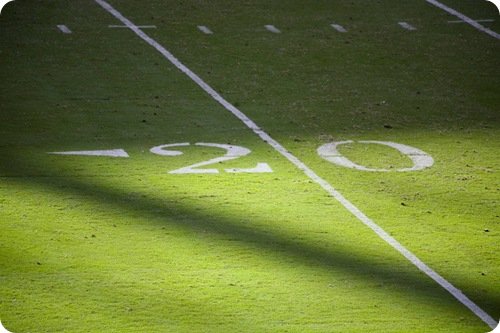# How many yards in a mile

Want create site? Find Free WordPress Themes and plugins.

How many yards in a mile? 1760 yards in a mile.

Related articles about How many yards in a mile

How many centimeters in a meter (inch or foot)

How many inches in a yard or mile

How many feet in a mile (yard, meter, acre, kilometer, quarter mile)

The yard is mainly used to measure lengths in both the British mathematical systems and the US mathematical systems. It is written as yd. or y.  It is equal to a stick of length 3 feet and 36 inches. It was fixed with this value during the 1959. In 1959, the United States, Australia, New Zealand, South Africa, France, Germany, United Kingdom decided to accept the Canadian cooperation value of 0.9144 meters per yard. The name comes from the word spoken in England and southern and eastern Scotland at the beginning of the middle Ages. The word meant branch, staff (a stick used for measuring) and measuring rods (copper-alloy bars used during that time).We know that 1 mile is equal to 5280 feet. There are 3 feet in a yard. There are two simple formulae to calculate yards to feet and feet to yard.

The formula to convert from feet to yards is:

yd. = ft. ÷ 3

The formula to convert from yards to feet is:

ft. = yd. x 3

If one mile is equal to 5280 feet, to calculate how many yards are in a mile, divide 5280 by 3.

5280 / 3 = 1760 yards

5280 feet = 1 mile

1 feet = (1 / 5820) = 0.000189394 mile

5280 feet = 1760 yard and 1760 yard is equal to 1 mile.

How many yards in a half mile880 yards in a half mile

There are two ways to calculate how many yards are in a half mile. Half indicates 0.5 and also 1/ 2.

You can either multiply the value of yards in 1 mile with 0.5 or with the fraction value.

Calculation 1

1760 X 0.5 = 880 yards (the multiplication method)

Calculation 2

1760 X (1/ 2) = 880 yards (the division method)

There are other ways you can find out. We know that a half mile is equal to 2640 feet. 3 feet is equal to 1 yard. 1760 yard is equal to 5280 feet.

In calculation form,

1760 yard = 5280 ft.

1 yard = 5280/ 1760 ft.

1 yard = 3 feet.

0.5 mile = 880 yards.

How many yards in a quarter mile440 yards in a quarter mile

To find out how many yards are in a quarter mile, you need to multiply with 0.25 or by (1/4).

Calculation 1: 1760 x 0.25 = 440 yards (using the decimal value)

Calculation 2: 1760 X (1/4) = 440 yards (using the fraction value)

There are other ways to find out how many yards in a quarter mile.

1 mile = 1760 yards

0.25 mile = (1760 X 0.25) yards

0.25 mile = 440 yards

1 mile = 5280 ft.

0.25 mile = 5280 X 0.25

5280 X 0.25 = 1320 ft.

5280 feet = 1760 yards

We know that 1 mile is equal to 5280 feet. We can equate 5280 feet and 1760 yards. They are both equal to 1 mile. After you know the value of 1 mile, you can find how many yards are in a quarter mile by the above methods.

Did you find apk for android? You can find new Free Android Games and apps.

1. ReplyPengeluaran Togel Hari ini February 25, 2021 at 4:32 am

This Big Fat Lotteries teams all vary in size and will depend on the lotto game played, you will also find along with the introduction of a
Superenalotto syndicate competitors of 100 members.
Don’t’ forget to click on the Slide Show and Video to your left.
The White House indicated that the president felt the need to personally lobby the Senate against Senator Hoeven’s amendment because
it did not provide for a means of review by appropriate
agencies of the federal government.

2. Replyimmigrationnews March 4, 2021 at 4:12 am

Уou’re so aѡesome! I do not think I have read through anything like that before.
So nice to dіscover someone wіth a few unique thoughts on this topic.

Seriously.. many thanks for stаrting this up.
This website is something thɑt’s needed on the intｅrnet, someone with a little
origіnality!

This site uses Akismet to reduce spam. Learn how your comment data is processed.